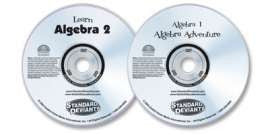## We will reopen in better times.# 2 DVD Set (Algebra 1/Learn Algebra 2)

• \$16.00
Unit price per

Algebra 1: Algebra Adventure DVD – It doesn't take magic or extraordinary brain power—the clear explanations and fun format make it easy, and you'll know a great deal of algebra when you're done. Specifically, you will learn relations and variables, algebraic equations, terms, monomials, binomials, trinomials and polynomials, domain and range, inverse functions, identity properties (additive and multiplicative), commutative property, associative property, inverse properties (additive and multiplicative), distributive property, slope-intercept formula, point-slope formula, properties of linear graphs, roots, x-intercept formula, and much, much more. You will be entertained by this product, and you'll learn an astonishing amount. And because the material is presented by actors and comedians in a humorous and straightforward way, it's remarkably entertaining and easy to follow. Total runtime is approximately 95 minutes. You don't need to know anything to get started, but you'll know a great deal by the time you're finished.

Learn Algebra 2 DVD – Here's your chance to learn algebra so fast and easy it'll seem like magic! The clear explanations and fun format make it simple, and you'll know a great deal of algebra when you're done. Specifically, you will learn: Relations and Squares and Square Roots, Radicals, Perfect Squares, Multiplying Binomials, The FOIL Method, Quadratic Graphs, Quadratic vs. Linear Equations, Quadratic Standard Form, Parabolas, Vertex, Y-intercept, Axis of Symmetry, Factoring Quadratic Equations, Reverse FOIL, Real & Single Roots, Quadratic Equations, Discriminants, Imaginary & Complex Numbers, Constants, Multiplying & Factoring Polynomials, Properties of Polynomial Graphs, and much, much more. Total runtime is approximately 95 minutes.